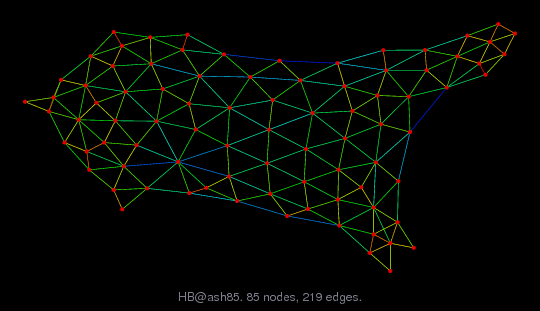Matrix: HB/ash85

Description: SYMMETRIC PATTERN OF NORMAL MATRIX OF HOLLAND SURVEY. ASHKENAZI, 1974(undirected graph drawing)• Matrix group: HB
• download as a MATLAB mat-file, file size: 1 KB. Use UFget(11) or UFget('HB/ash85') in MATLAB.

 Matrix properties number of rows 85 number of columns 85 nonzeros 523 structural full rank? yes structural rank 85 # of blocks from dmperm 1 # strongly connected comp. 1 explicit zero entries 0 nonzero pattern symmetry symmetric numeric value symmetry symmetric type binary structure symmetric Cholesky candidate? yes positive definite? no

 author V. Askenazi editor A. Curtis, I. Duff, J. Reid date 1974 kind least squares problem 2D/3D problem? no

 Ordering statistics: result nnz(chol(P*(A+A'+s*I)*P')) with AMD 505 Cholesky flop count 3.3e+03 nnz(L+U), no partial pivoting, with AMD 925 nnz(V) for QR, upper bound nnz(L) for LU, with COLAMD 598 nnz(R) for QR, upper bound nnz(U) for LU, with COLAMD 1,096

 SVD-based statistics: norm(A) 6.72155 min(svd(A)) 0.014494 cond(A) 463.746 rank(A) 85 sprank(A)-rank(A) 0 null space dimension 0 full numerical rank? yes

 singular values (MAT file): click here SVD method used: s = svd (full (A)) ; status: ok